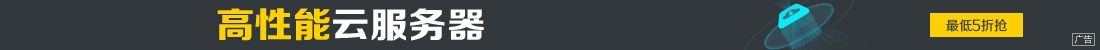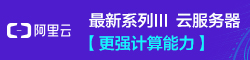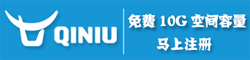# 深入解析Python中函数的参数与作用域

••• 参数的传递是通过自动将对象赋值给本地变量名来实现的。所有的参数实际上都是通过指针进行传递的，作为参数被传递的对象从来不自动拷贝。
• 在函数内部的参数名的赋值不会影响调用者。
• 改变函数的可变对象参数的值会对调用者有影响。

>>避免可变参数的修改

```L = [1,2]
changer(L[:])
```

```def changer(b):
b=b[:]
```

```L=[1,2]
changer(tuple(L))
```

>>对参数输出进行模拟

```def multiple(x,y):
x = 2
y = [2,4]
return x,y #Return new values in a tuple
```

>>基础知识

• 位置:从左至右进行匹配。
• 关键字参数:通过参数名进行匹配。(调用者可以定义哪一个函数接受这个值，通过在调用时使用参数的变量名，使用name=value这种语法。)
• 默认参数:为没有传入值的参数定义参数值。
• 可变参数:搜集任意多基于位置或关键字的参数。
• 可变参数解包:传递任意多的基于位置或关键字的参数。
• Keyword-only参数:参数必须按照名称传递。(只存在于Python3.0中)

>>匹配语法

func(value) 调用者 常规参数:通过位置进行匹配。
func(name=value) 调用者 关键字参数:通过变量名匹配。
func(*sequence) 调用者 以name传递所有的对象，并作为独立的基于位置的参数。
func(**dict) 调用者 以name成对的传递所有的关键字/值，并作为独立的关键字参数。
def func(name) 函数 常规参数:通过位置或变量名进行匹配。
def func(name=value) 函数 默认参数值，如果在调用中传递的话。
def func(*name) 函数 匹配并收集(在元组中)所有包含位置的参数。
def func(**name) 函数 匹配并收集(在字典中)所有包含位置的参数。
def func(*args,name) 函数 参数必须在调用中按照关键字传递。
def func(*,name=value) 函数 　参数必须在调用中按照关键字传递。(Python3.0)

>>细节

Python内部是使用以下的步骤来在赋值前进行参数匹配的:

• 通过位置分配非关键字参数。
• 通过匹配变量名分配关键字参数。
• 其他额外的非关键字分配到*name元组中。
• 其他额外的关键字参数分配到**name字典中。
• 用默认值分配给在头部未得到分配的参数。
• 在这之后，Python会进行检测，确保每个参数只传入了一个值。如果不是这样的话，将会发生错误。当所有匹配都完成了，Python把传递给参数名的对象赋值给它们。

>>关键字参数和默认参数的实例

```def f(a,b,c):
print(a,b,c)

f(1,2,3)   #Prints 1,2,3

```

```f(c=3,b=2,a=1) #Prints 1,2,3
```

```def f(a,b=2,c=3):
print(a,b,c)

f(1)    #Prints 1,2,3
f(1,4)   #Prints 1,4,3
f(1,c=6)   #Prints 1,2,6

```

```def func(spam,eggs,totast=0,ham=0):
print((spam,eggs,totast=0,ham=0))
func(1,2)     #Ouput:(1,2,0,0)
func(1,ham=1,eggs=0)  #Ouput:(1,0,0,1)
func(spam=1,eggs=0)   #Ouput:(1,0,0,0)
func(toast=1,eggs=2,spam=3) #Ouput:(3,2,1,0)
func(1,2,3,4)    #Ouput:(1,2,3,4)
```

>>任意参数的实例

```def f(*args):print(args)
```

**特性类似，但是它只对关键字参数有效。将这些关键字参数传递给一个新的字典，这个字典之后将能够通过一般的字典工具进行处理。在这种情况下，**允许将关键字参数转化为字典，你能够在之后使用键调用进行步进或字典迭代。

```def f(a,*pargs,**kargs):print(a,pargs,kargs)

f(1,2,3,x=1,y=2)  #Prints:1 (2,3) {'x':2,'y':1}

```

```def func(a,b,c,d):print(a,b,c,d)
args=(1,2)
args+=(3,4)
func(*args)   #Prints 1,2,3,4
```

```args={'a':1,'b':2,'c':3}
args['d']=4
func(**args)   #Prints 1,2,3,4
```

```if <test>:
action,args=func1,(1,)
else:
action,args=func2,(1,2,3)
...

action(*args)

```

>>Python3.0 Keyword-Only参数
Python3.0把函数头部的排序规则通用化了，允许我们指定keyword-only参数――即必须只按照关键字传递并且不会由一个位置参数来填充的参数。

```def kwonly(a,*,b,c):
print(a,b,c)
kwonly(1,c=3,b=2) #Prints:1,2,3
kwonly(c=3,b=2,a=1) #Prints:1,2,3
kwonly(1,2,3)  #Error!
```

```def kwonly(a,**pargs,b,c)  #Error!
def kwonly(a,**,b,c)   #Error!
```

```def f(a,*b,c=6,**d):print(a,b,c,d)

f(1,*(2,3),**dict(x=4,y=5))  #Prints:1 (2,3) 6 {'x':4,'y':5}
f(1,*(2,3),**dict(x=4,y=5),c=7) #Error!
f(1,*(2,3),c=7,**dict(x=4,y=5)) #Prints:1 (2,3) 7 {'x':4,'y':5}
f(1,c=7,*(2,3),**dict(x=4,y=5)) #Prints:1 (2,3) 7 {'x':4,'y':5}
f(1,*(2,3),**dict(x=4,y=5,c=7)) #Prints:1 (2,3) 7 {'x':4,'y':5}

```

Python作用域

def之中的变量名与def之外的变量名并不冲突，一个在def之外被赋值的变量X与在这个def之中赋值的变量X是完全不同的变量。
>>作用域法则

>>变量名解析:LEGB原则
Python的变量名解析机制有时称为LEGB法则，当在函数中使用未认证的变量名时，Python搜索4个作用域:

• 本地作用域(L)
• 上一层结构中def或lambda的本地作用域(E)(其实就是函数嵌套的情况)
• 全局作用域(G)
• 最后是内置作用域(B)

Python按顺序在上面4个作用域中查找变量，并且在第一个能够找到这个变量名的地方停下来，如果在这4个作用域中都没找到，Python会报错。

```s=10
def times(x,y):
x=s
return x*y

times(3,4) #return 40 not 12

```

>>内置作用域

```import builtins
dir(builtins)
```

global语句

global语句是一个命名空间的声明，它告诉Python解释器打算生成一个或多个全局变量，也就是说，存在于整个模块内部作用域(命名空间)的变量名。关于全局变量名:

global语句包含了关键字global，其后跟着一个或多个由逗号分开的变量名。当在函数主题被赋值或引用时，所有列出来的变量名将被映射到整个模块的作用域内。 举个例子:

```X=88
def func():
global X
X = 99

func()
print(X) #Prints 99

```

>>嵌套作用域的细节

>>嵌套作用域举例

```X = 99
def f1():
X = 88
def f2():
print(X)
f2()
f1() #Prints 88:enclosing def local
```

```X = 99
def f1():
X = 88
def f2():
print(X) #Remember X in enclosing def scope
return f2 #Return f2 but don't call it

action = f1() #Make return function
action() #Call it now:Prints 88

```

```def maker(N):
def action(X):
return X ** N
return action

f=maker(2) #Pass 2 to N
f(3) #Pass 3 to X,N remembers 2: 3**2,Return 9
f(4) #return 4**2

g=maker(3) #g remembers 3,f remembers 2
g(3) #return 27
f(3) #return 9

```

```def makeActions():
acts=[]
for i in range(5): #Tries to remember each i
acts.append(lambda x: i ** x) #All remember same last it
return acts
```

```def makeActions():
acts=[]
for i in range(5): #Use default instead
acts.append(lambda x,i=i: i ** x) #Remember current i
return acts
{
```

nonlocal语句

>>nonlocal基础

```def func():
nonlocal name1,name2...
```

global使得作用域查找从嵌套的模块的作用域开始，并且允许对那里的名称赋值。如果名称不存在与该模块中，作用域查找继续到内置作用域，但是，对全局名称的赋值总是在模块作用域中创建或修改它们。
nonlocal限制作用域查找只是嵌套的def，要求名称已经存在于那里，并且允许对它们赋值。作用域查找不会继续到全局或内置作用域。
>>nonlocal应用

```def tester(start):
state = start #each call gets its own state
def nested(label):
nonlocal state #remember state in enclosing scope
print(label,state)
state+=1 #Allowed to change it if onolocal
return nested

F = tester(0) #Increments state on each call
F('spam') #Prints:spam 0
F('ham') #Prints:ham 1
F('eggs') #Prints:eggs 2

```

nonlocal限制作用域查找仅为嵌套的def，nonlocal不会在嵌套的模块的全局作用域或所有def之外的内置作用域中查找。